# Two Point Form

In this mini-lesson, you will learn about the two-point form of a line.

The equation of a line can be found through various methods depending on the available information.

The two-point form is one of the methods.

This is used to find the equation of a line when two points are given.

You can explore the two-point form using the following two-point form calculator.

Enter any two points on the line to get its equation using the two-point form.

## Lesson Plan

 1 What Is the Equation of a Line in Two-Point Form? 2 Important Notes on Two-Point Form 3 Solved Examples on Two-Point Form 4 Thinking Out of the Box! 5 Interactive Questions on Two-Point Form

## What Is the Equation of a Line in Two-Point Form?

The equation of a line represents each and every point on the line, i.e., it is satisfied by each point on the line.

The two-point form of a line is used for finding the equation of a line given two points $$(x_1,y_1)$$ and $$(x_2,y_2)$$ on it.

The two-point form of a line passing through these two points is:

 $y-y_1= \dfrac{y_2-y_1}{x_2-x_1}(x-x_1)$(or)$y-y_2= \dfrac{y_2-y_1}{x_2-x_1}(x-x_2)$

Here, $$(x, y)$$ represents any random point on the line and we keep $$x$$ and $$y$$ as variables.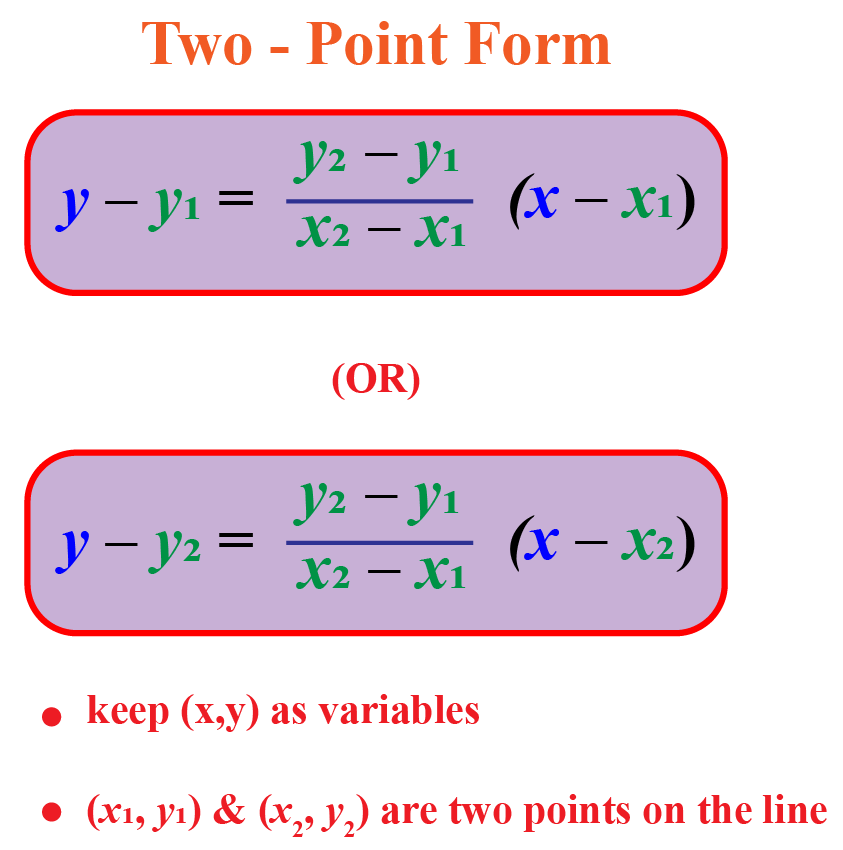## How Do You Find the Equation of a Line That Passes Through Two Points?

How do we derive the two-point form of a line? Let's see.

Let us consider two fixed points $$A\,(x_1,y_1)$$ and $$B\,(x_2,y_2)$$ on the line.

Let us assume that $$C\,(x, y)$$ is any random point on the line.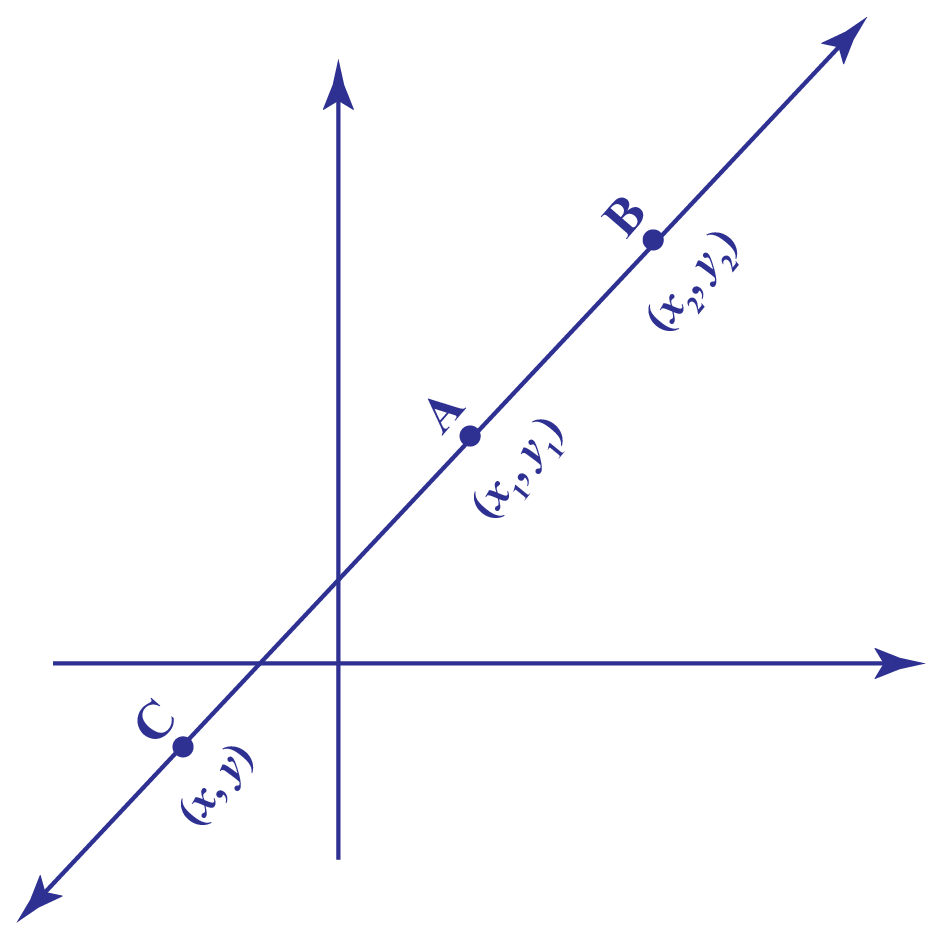Since $$A, B$$, and $$C$$ lie on the same line:

Slope of $$\overleftrightarrow{AC}$$ = Slope of $$\overleftrightarrow{AB}$$

Using the two point slope formula,

\begin{align} \dfrac{y-y_1}{x-x_1}&= \dfrac{y_2-y_1}{x_2-x_1}\\[0.2cm] \text{Multiplying both }& \text{ sides by }(x-x_1),\\[0.2cm] y-y_1&= \dfrac{y_2-y_1}{x_2-x_1}(x-x_1) \end{align}

Hurray! We derived the two-point form. It is used to find the equation of a line that passes through two points.

Can you try deriving another formula of two-point form, which is, $$y-y_2= \dfrac{y_2-y_1}{x_2-x_1}(x-x_2)$$ now?

## Solved Examples

 Example 1

Can we help Mary find the equation of a straight line passing through the points A (1, 2) and B (-1, 3)?

Solution

The following figure shows the line passing through the given points: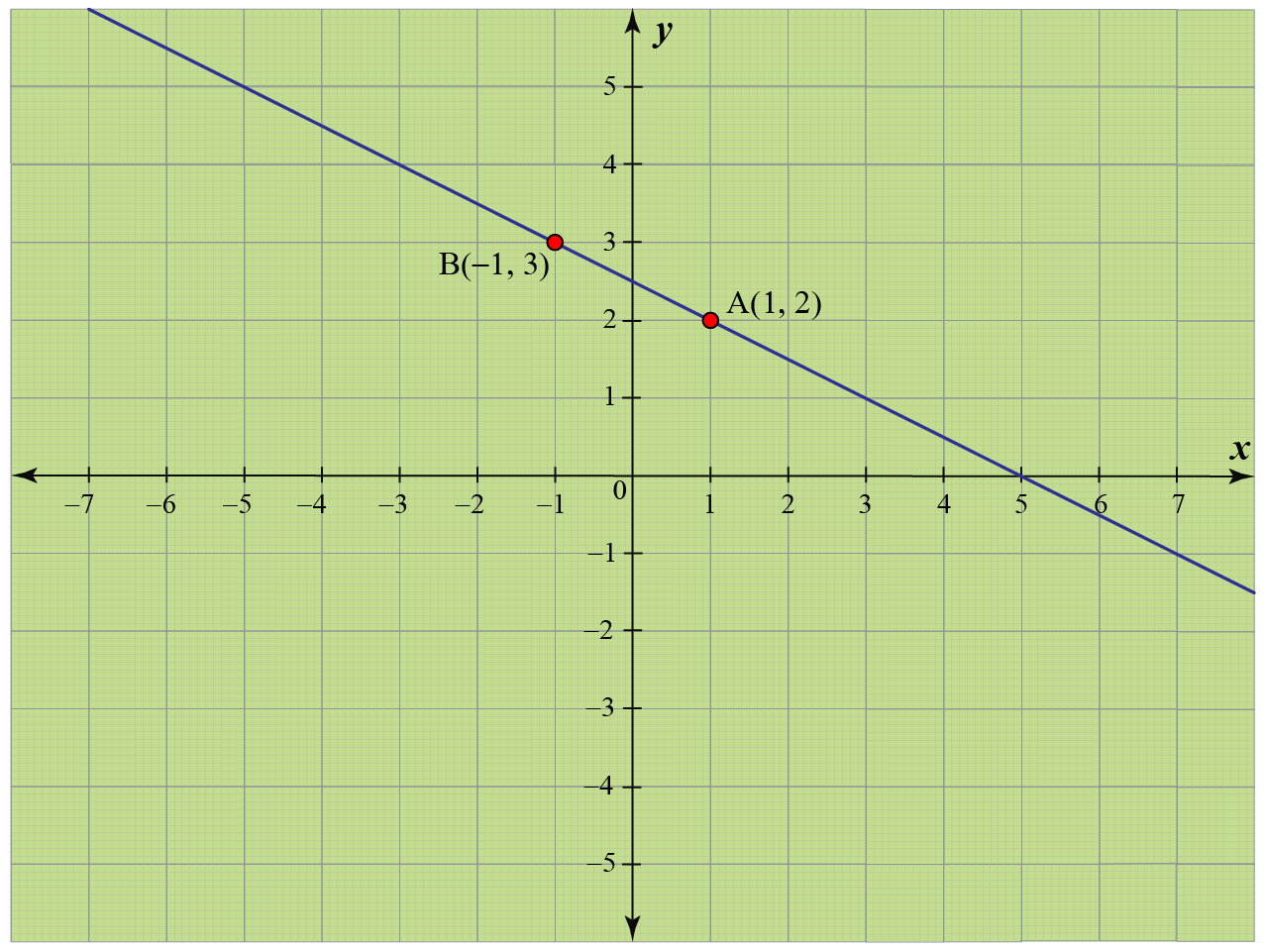The given two points are: $A(1,2)=(x_1,y_1)\\[0.2cm] B(-1,3)= (x_2, y_2)$

Since we know two points on the line, we use the two-point form to find its equation.

\begin{align}y-y_1&= \dfrac{y_2-y_1}{x_2-x_1}(x-x_1) \\[0.2cm] y-2 &= \dfrac{3-2}{-1-1}(x-1) \\[0.2cm] y-2 &= \dfrac{1}{-2}(x-1)\\[0.2cm] \text{Multiplying } &\text{both sides by -2},\\[0.2cm] -2(y-2) &= x-1\\[0.2cm] -2y+4 &= x-1\\[0.2cm] x+2y&-5=0 \end{align}

Therefore, the equation of the line is,

 $$\therefore$$ $$x+2 y-5=0$$
 Example 2

Can we help Margarette find the y-intercept of the line passing through the points A (3, -2) and B (-1, 3)?

Solution

The following figure shows the line passing through the given points: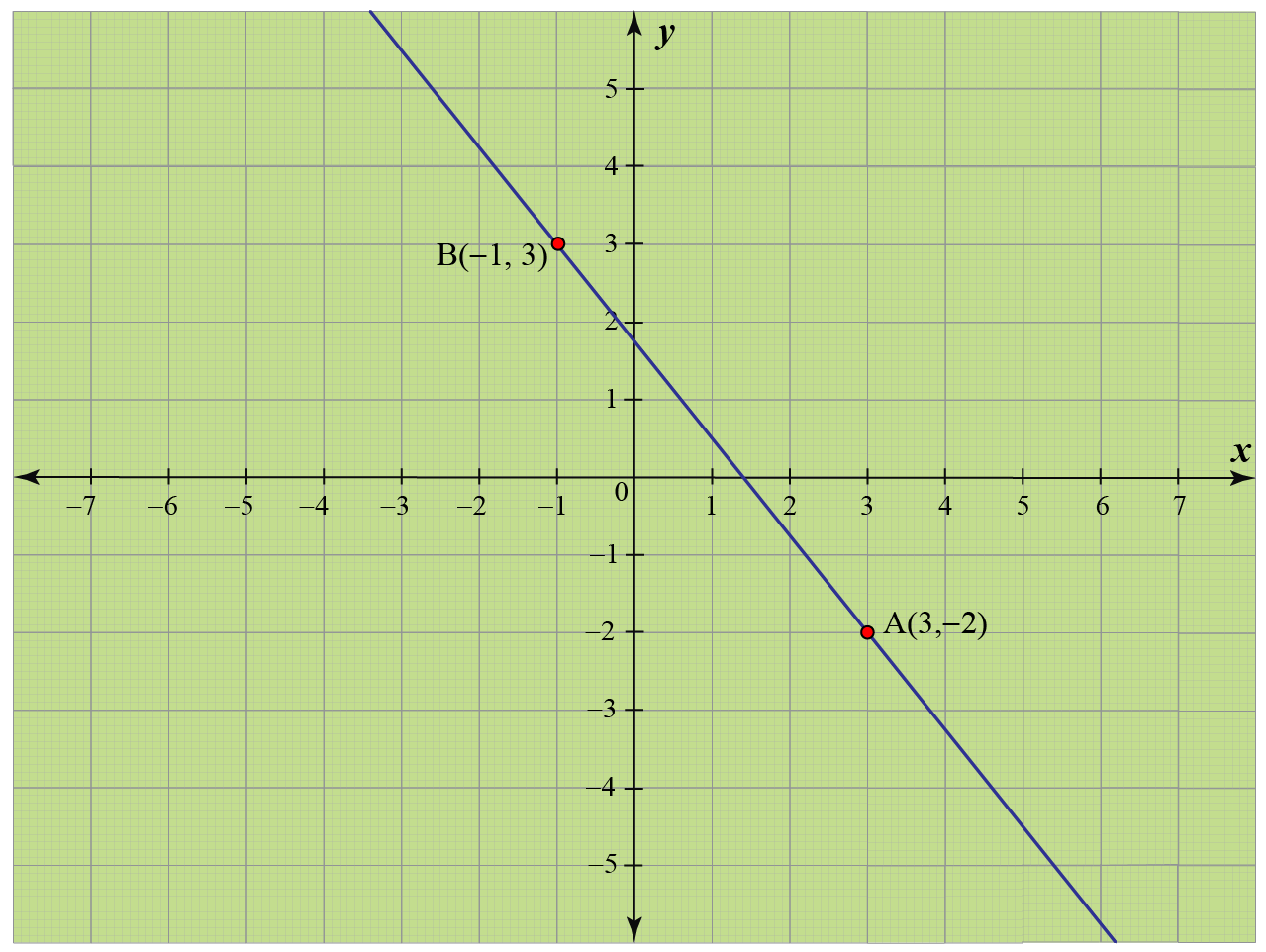The given two points are: $A(3,-2)=(x_1,y_1)\\[0.2cm] B(-1,3)= (x_2, y_2)$

Since we know two points on the line, we use the two-point form to find its equation.

\begin{align}y-y_1&= \dfrac{y_2-y_1}{x_2-x_1}(x-x_1) \\[0.2cm] y+2 &= \dfrac{3+2}{-1-3}(x-3) \\[0.2cm] y+2 &= \dfrac{5}{-4}(x-3)\\[0.2cm] \text{Multiplying } &\text{both sides by -4},\\[0.2cm] -4(y+2) &= 5(x-3)\\[0.2cm] -4y-8 &= 5x-15\\[0.2cm] -4y&= 5x-7\\[0.2cm] y&=\left(-\frac{5}{4}\right) x+\frac{7}{4}\end{align}

The final equation is in the slope-intercept form, $$y=mx+b$$.

Comparing the last two equations, we get the y-intercept to be

 $$\therefore$$ $$b=\dfrac{7}{4}$$
 Example 3

Find the equation of a straight line whose x-intercept is $$a$$ and y-intercept is $$b$$, as shown in the following figure.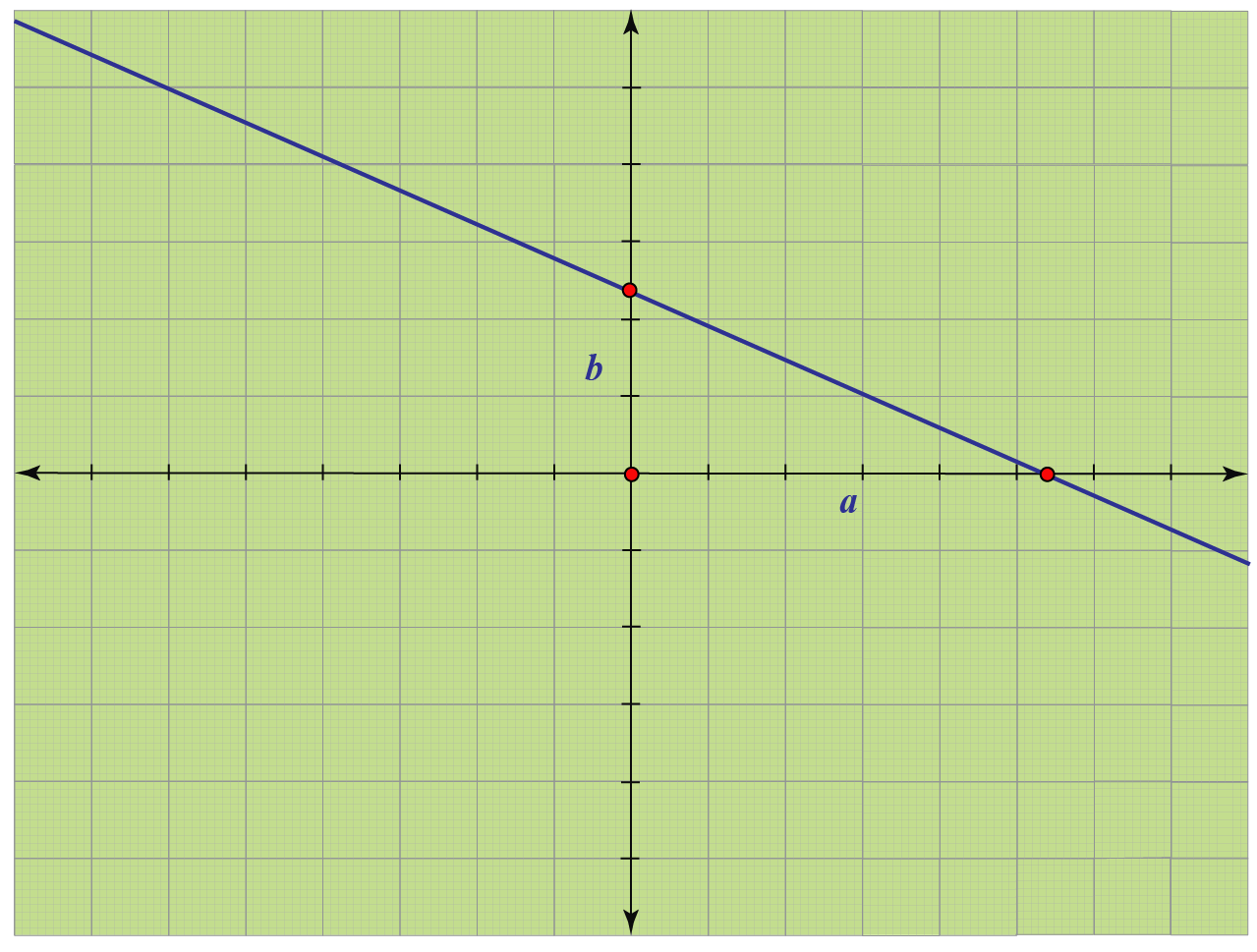Solution

The given line passes through the points: $A(a, 0)=(x_1,y_1)\\[0.2cm] B(0, b)= (x_2, y_2)$

Since we know two points on the line, we use the two-point form to find its equation.

\begin{align}y-y_1&= \dfrac{y_2-y_1}{x_2-x_1}(x-x_1) \\[0.2cm] y-0&= \dfrac{b-0}{0-a}(x-a) \\[0.2cm] y&= \dfrac{b}{-a}(x-a)\\[0.2cm] \text{Multiplying } &\text{both sides by }-a,\\[0.2cm] -ay &= b(x-a)\\[0.2cm] -ay&=bx-ab\\[0.2cm] bx+ay&=ab \\[0.2cm] \text{Dividing } &\text{both sides by }ab,\\[0.2cm] \frac{x}{a}+\frac{y}{b}&=1\end{align}

Thus, the equation of the given line is:

 $$\therefore$$ $$\frac{x}{a}+\frac{y}{b}=1$$

Note: This is also called the intercept-form of a line.Think Tank
1. Which of the following graphs represents the equation $$y-2=\dfrac{1-2}{-2-1}(x-1)$$?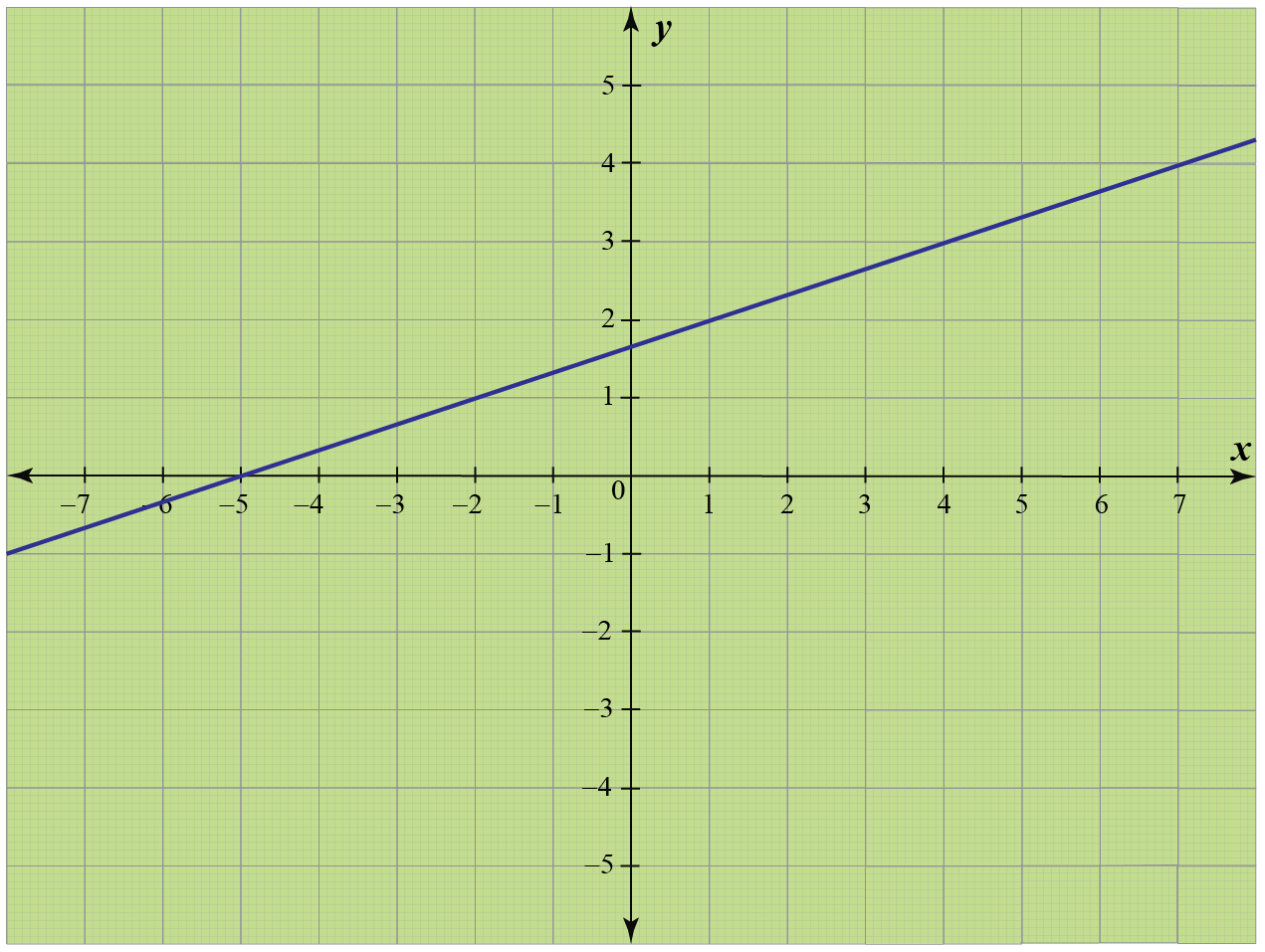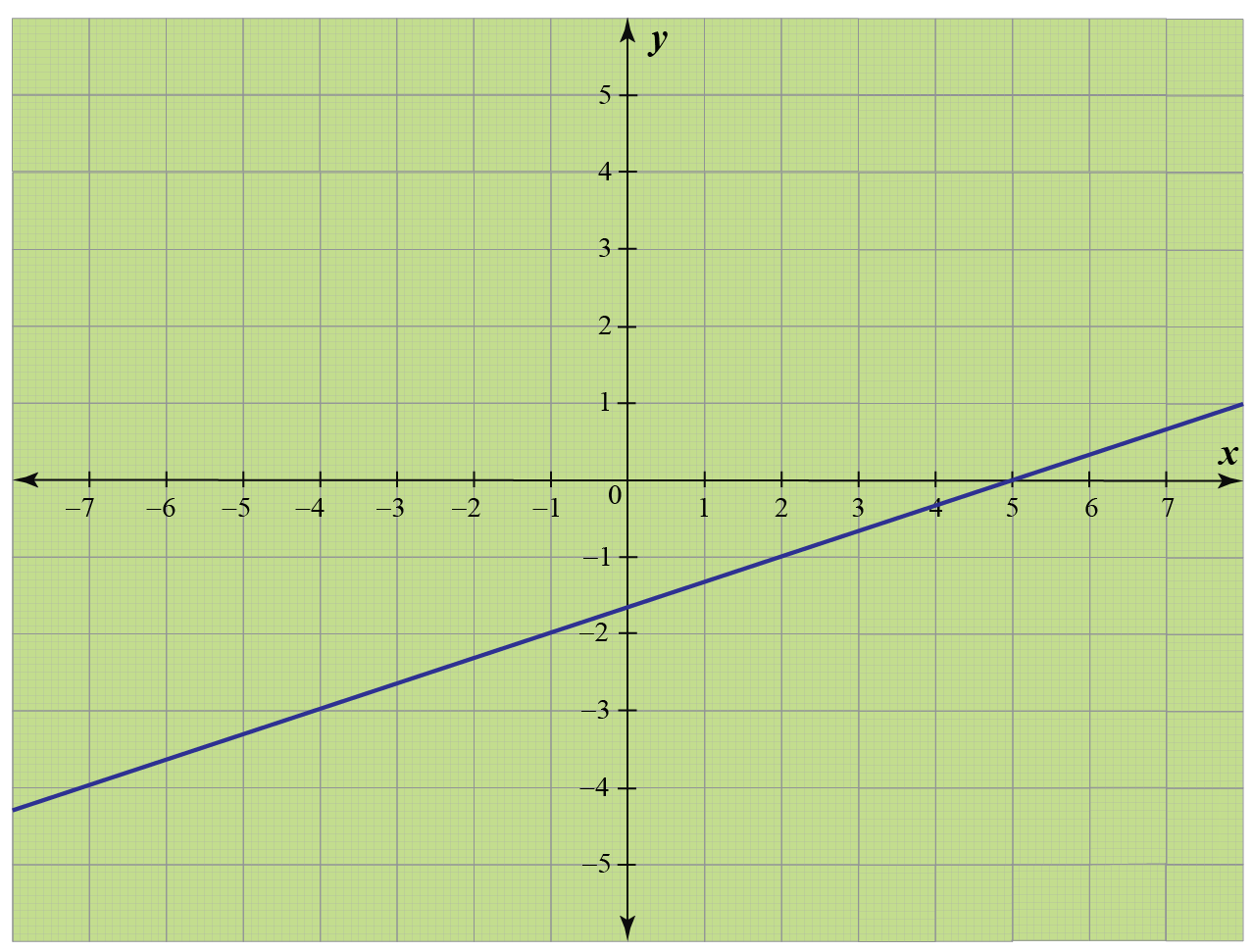## Interactive Questions

Here are a few activities for you to practice.

## Let's Summarize

The mini-lesson targeted the fascinating concept of Two-Point Form. The math journey around Two-Point Form starts with what a student already knows, and goes on to creatively crafting a fresh concept in the young minds. Done in a way that not only it is relatable and easy to grasp, but also will stay with them forever. Here lies the magic with Cuemath.

At Cuemath, our team of math experts is dedicated to making learning fun for our favorite readers, the students!

Through an interactive and engaging learning-teaching-learning approach, the teachers explore all angles of a topic.

Be it worksheets, online classes, doubt sessions, or any other form of relation, it’s the logical thinking and smart learning approach that we, at Cuemath, believe in.

## FAQs on Two-Point Form

### 1. What is the two-point form?

The two-point form of a line is used for finding the equation of a line given two points $$(x_1,y_1)$$ and $$(x_2,y_2)$$ on it.

The two point-form of a line is:$y-y_1= \dfrac{y_2-y_1}{x_2-x_1}(x-x_1)$ $\text{(or)}$$y-y_2= \dfrac{y_2-y_1}{x_2-x_1}(x-x_2)$

### 2. How do you write an equation with two given points?

We substitute the points in the two-point form to find the equation.

### 3. How do you find the intercept form?

The intercept form of a line whose and intercepts are $$a$$ and $$b$$ respectively is $$\frac{x}{a}+\frac{y}{b}=1$$.

To know how to find this, refer to Example 3 under the "Solved Examples" section of this page.

### 4. What is the example of a point slope form?

The equation of a line with slope, $$m= 1$$ that passes through a point $$(x_1,y_1)= (-2, 3)$$ using the point-slope form is:

\begin{align} y-y_1&=m(x-x_1)\\[0.2cm] y-3&=1(x+2) \\[0.2cm] y&=x+5 \end{align}

### 5. What is the Y intercept in an equation?

To find the y-intercept in an equation, substitute x = 0 and solve for y.

### 6. How do you find the Y intercept with two points and slope?

• First, find the equation of the line using the two-point form and solve it for $$y$$.
• Compare it with $$y=mx+b$$
• Here, $$b$$ is the y-intercept.

### 7. What is the normal form of a line?

The normal form of a line is $$x \cos \alpha+y \sin \alpha=p$$.

Here, $$\alpha$$ is the angle made by the line with the positive direction of the x-axis and

$$p$$ is the perpendicular distance of the line from the origin.

### 8. How do you find the equation of a line with only one point?

We can't find the equation of a line just with one point.

To find the equation of a line one of the following information should be available.

• Two points on a line.
• One point and the slope of a line.
• One point and one intercept of a line.
• Two intercepts of a line.

### 9. How do you determine if a point lies on a line?

Every point on a line satisfies its line equation.

For example, to see whether (1, 2) lies on a line $$y= 2x$$, we substitute $$x=1$$ and $$y=2$$ in the given equation. Then we get:

$2= 2(1)\\[0.1cm] 2=2$

The equation is satisfied and hence the point (1, 2) lies on the line $$y=2x$$.

### 10. How do you find the slope of a line with two given points?

To find the slope of a line with two given points:

• First find the equation of line using the two-point form and solve it for $$y$$.
• Compare it with $$y=mx+b$$
• Here, $$m$$ is the slope of the line.
Coordinate Geometry
Grade 9 | Questions Set 1
Coordinate Geometry
Coordinate Geometry
Grade 10 | Questions Set 1
Coordinate Geometry